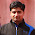## Search within website

### Sample paper 3

1.  What are wind energy farms?

2.  What is meant by potential difference between two points?

3.  How much energy will an electric bulb draw from a 220 V source, if the resistance of the bulb-filament is 1200 ohms?

4. What is electromagnetic induction?

5. In what way can the magnitude of induced current be increased?

6. Calculate the electrical energy consumed by a 1200 W toaster in 20 minutes.

7. Biomass has been used as fuel since ancient times, how has it been modified to function as a more efficient fuel in the recent past.

8. Two identical resistors, each of resistance 20 ohms are connected (i) in parallel (ii) in series, in turn, to a battery of 10V. Calculate the ratio of power consumed in the combination of resistors in the two cases.

9. Two resistors of resistances 3 ohms and 6 ohms respectively are connected to a battery of 6 V so as to have
(a)    Minimum resistance,    (b) minimum current
(i)  How will you connect the resistances in each case?
(ii) Calculate the strength of the current in the circuit in both cases.

10. Explain what is short circuiting and overloading in an electric supply?

11. Why can’t two magnetic field lines cross each other?

12. Draw the magnetic field lines (including field directions) of the magnetic field due to a long straight solenoid. What important property of this field is indicated by this field line pattern? Name any two factors on which the magnitude of the magnetic field due to this solenoid depends.

13. State the rule to determine the direction of a
(i)  Magnetic field produced around a straight conductor carrying current.
(ii) Force experienced by current – carrying straight conductor placed in a magnetic field which is perpendicular to it.
(iii) Current induced in a coil due to its rotation in a magnetic field.

14. Differentiate between AC and DC. Write one advantage of AC over DC.

Other Sample Paper in this blog:

3.   Sample Paper 3 (currently opened)

1.1.